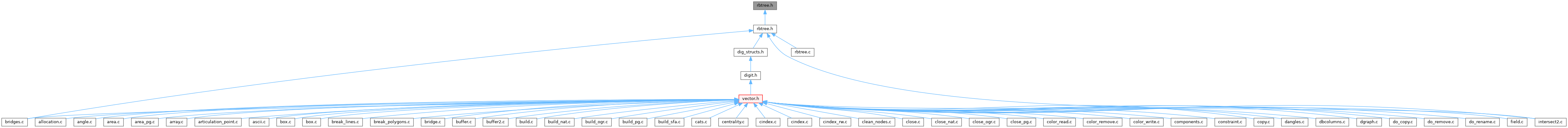GRASS GIS 8 Programmer's Manual  8.2.2dev(2023)-5c00b4e944
defs/rbtree.h File Reference
This graph shows which files directly or indirectly include this file:Go to the source code of this file.

## Functions

struct RB_TREErbtree_create (rb_compare_fn *, size_t)

void rbtree_clear (struct RB_TREE *)

void rbtree_destroy (struct RB_TREE *)

int rbtree_insert (struct RB_TREE *, void *)

int rbtree_remove (struct RB_TREE *, const void *)

void * rbtree_find (struct RB_TREE *, const void *)

int rbtree_init_trav (struct RB_TRAV *, struct RB_TREE *)

void * rbtree_traverse (struct RB_TRAV *)

void * rbtree_traverse_backwd (struct RB_TRAV *trav)

void * rbtree_traverse_start (struct RB_TRAV *, const void *)

int rbtree_debug (struct RB_TREE *, struct RB_NODE *)

## ◆ rbtree_clear()

 void rbtree_clear ( struct RB_TREE * tree )

Definition at line 490 of file rbtree.c.

References RB_NODE::data, free(), RB_NODE::link, NULL, and RB_TREE::root.

Referenced by rbtree_destroy().

## ◆ rbtree_create()

 struct RB_TREE* rbtree_create ( rb_compare_fn * compare, size_t rb_datasize )

Definition at line 50 of file rbtree.c.

## ◆ rbtree_debug()

 int rbtree_debug ( struct RB_TREE * tree, struct RB_NODE * root )

Definition at line 530 of file rbtree.c.

## ◆ rbtree_destroy()

 void rbtree_destroy ( struct RB_TREE * tree )

Definition at line 520 of file rbtree.c.

References free(), NULL, and rbtree_clear().

## ◆ rbtree_find()

 void* rbtree_find ( struct RB_TREE * tree, const void * data )

Definition at line 243 of file rbtree.c.

## ◆ rbtree_init_trav()

 int rbtree_init_trav ( struct RB_TRAV * trav, struct RB_TREE * tree )

Definition at line 264 of file rbtree.c.

References assert, RB_TRAV::curr_node, RB_TRAV::first, RB_TREE::root, RB_TRAV::top, and RB_TRAV::tree.

## ◆ rbtree_insert()

 int rbtree_insert ( struct RB_TREE * tree, void * data )

Definition at line 74 of file rbtree.c.

## ◆ rbtree_remove()

 int rbtree_remove ( struct RB_TREE * tree, const void * data )

Definition at line 154 of file rbtree.c.

## ◆ rbtree_traverse()

 void* rbtree_traverse ( struct RB_TRAV * trav )

Definition at line 281 of file rbtree.c.

References assert, RB_TRAV::curr_node, RB_TRAV::first, G_debug(), and NULL.

## ◆ rbtree_traverse_backwd()

 void* rbtree_traverse_backwd ( struct RB_TRAV * trav )

Definition at line 307 of file rbtree.c.

References assert, RB_TRAV::curr_node, RB_TRAV::first, G_debug(), and NULL.

## ◆ rbtree_traverse_start()

 void* rbtree_traverse_start ( struct RB_TRAV * trav, const void * data )

Definition at line 335 of file rbtree.c.